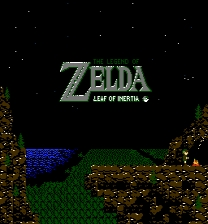Parameter Info
Console: NES
Type: Complete
Genre: Role Playing > Action RPG
Modifications: L,GP
Creator: the_icepenguin
Date Created: 01/20/2011
Parameter Info
File Name: Zelda II - Leaf of Inertia v1-2.zip
Requirements: No Special Requirements
Version: 1.2
Rating:

Zelda II - Leaf Of InertiaDescription

Don’t think this is gonna be a 100% Gold Remake, it’ll have its differences. Extra battles (some’ll be optionals, tough but rewarding), Hoenn Pokemon (in every area, and hard to find) New areas, Remapped some dungeons (so your old guides wont work) , Extra recurring characters (some’ll hate you, some’ll help you)

Note that the hack is incomplete but feel free to enjoy the hack as-is.

Zelda II - Leaf Of InertiaRead Me

_                               _          __   ______    _     _
| |    The                      | |        / _| |___  /   | |   | |
| |     ___  __ _  ___ _ __   __| |   ___ | |_     / / ___| | __| | __ _
| |    / _ \/ _` |/ _ \ '_ \ / _` |  / _ \|  _|   / / / _ \ |/ _` |/ _` |
| |___|  __/ (_| |  __/ | | | (_| | | (_) | |    / /_|  __/ | (_| | (_| |
|______\___|\__, |\___|_| |_|\__,_|  \___/|_|   /_____\___|_|\__,_|\__,_|
__/ |
|___/          "Leaf of Inertia"

=-*=-*=-*=-*=-*=-*=-

Hack By: Ice Penguin  (Doug Cengiz)
Version: 1.2
Email: the_icepenguin@yahoo.com

Started: 1-01-11 | Release Date: 1-28-11 | Last Update: 1-30-11

=-*=-*=-*=-*=-*=-*=-*=-*=-*=-*=-*=-*=-*=-*=-*=-*=-*=-*=-*=-*=-*=-*=-*=-*=-*=-*=
=-*=-*=-*=-*=-*=-*=-*=-*=-*=-*=-*=-*=-*=-*=-*=-*=-*=-*=-*=-*=-*=-*=-*=-*=-*=-*=

I)   Introduction to the Hack

II)  How to Play this Hack

III) Version History

IV)  Credits

V)   Contact Me

=-*=-*=-*=-*=-*=-*=-*=-*=-*=-*=-*=-*=-*=-*=-*=-*=-*=-*=-*=-*=-*=-*=-*=-*=-*=-*=
I) Introduction to the Hack
=-*=-*=-*=-*=-*=-*=-*=-*=-*=-*=-*=-*=-*=-*=-*=-*=-*=-*=-*=-*=-*=-*=-*=-*=-*=-*=

This hack was based off my Journey of a Day hack, so it didn't take long to
complete.

It's the original game but with a twist.  Link can't jump.  While the world
map and dungeon layouts are mostly unchanged, I have designed them with no
jumping in mind.

I won't spoil anymore, but just play it for yourself.  :)

EASY MODE - Enemies do 1x damage to Link.

HARD MODE - Enemies do 2x damage to Link.

=-*=-*=-*=-*=-*=-*=-*=-*=-*=-*=-*=-*=-*=-*=-*=-*=-*=-*=-*=-*=-*=-*=-*=-*=-*=-*=
II) How to Play this Hack
=-*=-*=-*=-*=-*=-*=-*=-*=-*=-*=-*=-*=-*=-*=-*=-*=-*=-*=-*=-*=-*=-*=-*=-*=-*=-*=

Use the "Zelda 2 - The Adventure of Link (U)" ROM for this hack.

Not all emulators will play my hack.  So check out the list below and Google
the one you want to use.  There might be later versions of each emulator, not
too sure, but I listed these specific versions, because they play my hack best.

Emulator:         Version:
~~~~~~~~~         ~~~~~~~~
FCE Ultra         98.12
FCEUXD            1.06
Jnes              0.6
Nestopia          1.37

For intended quality, I would suggest using Jnes.  I prefer it. ;)

Also, there may be some other emulators that will play my hack, but I could
only test these ones.

Patching the ROM:
~~~~~~~~~~~~~~~~~
To do this, you can use FuSoYa's Lunar IPS tool.  It's very easy to use, so you
shouldn't have too much trouble.  Here is the website:

http://fusoya.eludevisibility.org/projects.html

=-*=-*=-*=-*=-*=-*=-*=-*=-*=-*=-*=-*=-*=-*=-*=-*=-*=-*=-*=-*=-*=-*=-*=-*=-*=-*=
III) Version History
=-*=-*=-*=-*=-*=-*=-*=-*=-*=-*=-*=-*=-*=-*=-*=-*=-*=-*=-*=-*=-*=-*=-*=-*=-*=-*=

January 01, 2011 -
~~~~~~~~~~~~~~~~~~
- I started the hack.

January 28, 2011 - Version 1.0
~~~~~~~~~~~~~~~~~~
- Release date of my hack.

January 29, 2011 - Version 1.1
~~~~~~~~~~~~~~~~~~
- Just a small fix.  I completely forgot about a magic container being in
a certain spot.  :p  It had to be moved.

January 30, 2011 - Version 1.2
~~~~~~~~~~~~~~~~~~
- Polished everything up!  Made a few battle encounters in East Hyrule
easier.
- Added an extra life to both versions.  Increased experience gains on a
few monsters.
- Added an extra version with jump enabled.  :)

=-*=-*=-*=-*=-*=-*=-*=-*=-*=-*=-*=-*=-*=-*=-*=-*=-*=-*=-*=-*=-*=-*=-*=-*=-*=-*=
IV) Credits
=-*=-*=-*=-*=-*=-*=-*=-*=-*=-*=-*=-*=-*=-*=-*=-*=-*=-*=-*=-*=-*=-*=-*=-*=-*=-*=

Nintendo:
I'm glad they made Zelda II.  One of my favorite games.  :)

Acmlm's Board/Board2:
Awesome online community.  Where my ROM hacking was started.
http://acmlm.no-ip.org/board/

Ice Penguin:

JaSp:
He helped me out A LOT while making this hack!  Not only did he teach me
quite a bit about ASM, but he also contributed!  He did the Overworld
animation, and more...

Dwedit:
He made the level editor I used. If you want to try it out, you can download
it on his website: http://dwedit.home.comcast.net/

Trax:
Taught me a lot about hacking.  ASM and stuff.  He even contributed, as well!

Ice Ranger:
Helped find data information in the ROM.

You:
For playing it!  Yay!

=-*=-*=-*=-*=-*=-*=-*=-*=-*=-*=-*=-*=-*=-*=-*=-*=-*=-*=-*=-*=-*=-*=-*=-*=-*=-*=
V) Contact Me
=-*=-*=-*=-*=-*=-*=-*=-*=-*=-*=-*=-*=-*=-*=-*=-*=-*=-*=-*=-*=-*=-*=-*=-*=-*=-*=

Please send me an email, if you have any questions or comments, or if you find
any bugs or glitches in my hack:

the_icepenguin@yahoo.com

=-*=-*=-*=-*=-*=-*=-*=-*=-*=-*=-*=-*=-*=-*=-*=-*=-*=-*=-*=-*=-*=-*=-*=-*=-*=-*=